# Convergence of non-linear calculations

## Convergence & Divergence#

The nonlinear calculation is an iterative calculation. The term ‘convergence’ means the property of results to go for a certain value with every iteration step. If this is not the case (results alternate or show increasing differences to the previous iteration step), it is labeled ‘divergence.’ In these cases, the calculation does not produce any results.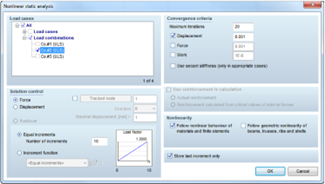The criteria for convergence can be set in the parameters for non-linear calculations. In most cases, it is recommended to use the displacement as a convergence criterion. The value of the convergence criteria should only be modified with a thorough understanding of the calculation procedure since it does not just influence the necessary number of iteration steps (and therefore the calculation time) but the accuracy of results as well.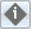During calculation, the convergence behavior can be viewed under “iterations.” After the calculation, it can be reviewed under “model information” (vertical toolbar, left).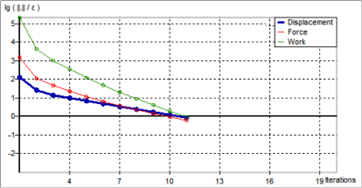Convergence is reached if the curve of the criteria in use (bold) drops below zero.

If the curve does not drop below zero, divergence is assumed.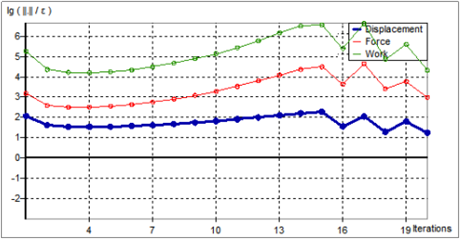• Curve drops, but not below zero
• Curve is (more or less) constant
• Curve alternates around a certain value
• Curve rises (after a certain increment)

Different situations can cause divergence. Appropriate steps can be determined based on the model and the convergence curve.

## Stability under non-linear circumstances#

Instabilities can occur in a non-linear calculation, even if the model is stable under linear conditions.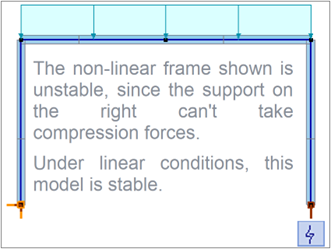• Non-linear element properties prevent equilibrium (see example on the right)
• High load lead to increasing deformations (e.g., exceeding a columns buckling force)

Non-linear instabilities cause the convergence curve to rise and produce an error after the calculation. In some cases, the calculation can be completed, but the results clearly violate the non-linear element properties (e.g., the tension in a support with the exclusion of tension).

### Maximum Iterations#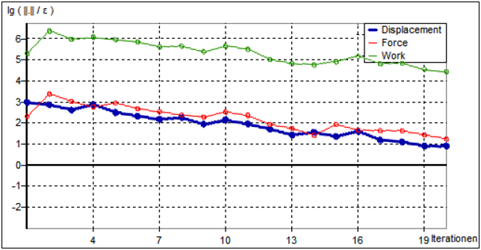If the convergence curve drops without dropping below zero, it can be assumed that the calculation does converge but that a greater number of iteration steps is needed. The “maximum iterations” can be set to a greater value in these cases. Remark | the reduction of maximum iterations for converging systems is only going to reduce calculation time if the calculation is not converging to the criteria, because the calculation is automatically stopped when the convergence criteria is met, even if it means that less than the maximum number of iterations has been calculated.

### Secant Stiffness#

Plastic hinges can cause an alternating behavior around the beginning plastification. The convergence curve will alternate around a constant (positive) value.

As a first step, it should be tried to run the calculation with increased (equal) increments (e.g., 30, 50, 100). If this does not lead to convergence, the option “use secant stiffness” can be selected. When doing so, the number of maximum iterations should be increased (> 100) since these calculations typically converge slowly. In some cases, using a user-defined “increment function” (see below) can lead to a successful calculation.

### Number of increments & Increment function#If divergence is caused by geometric non-linearities (big deformations in individual increments), a user-defined increment function can be used to run the calculation. As a first step, an increased number of “equal increments” (linear increment function) should be used since developing a suitable increment function can be rather time-consuming.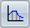The increment function is defined by a series of support points in the table of the “increment function editor.” The values are sorted automatically by the increment number N.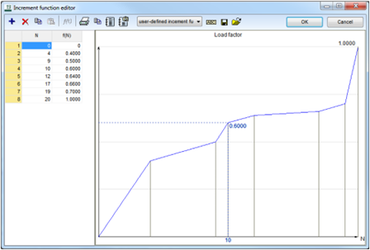To consider non-linear material and/or element properties, the increment function has to rise continuously (no horizontal or dropping sections).

The calculation of geometric non-linearities (influence of the deformation on the internal forces) can be done with any increment function.

### Development of the increment function#

Both procedures described below follow some common principles.

• The iterative procedure with multiple calculation runs
• Calculation with an increased number of “equal increments” as a first step
• Modification of the increment function between the last converging increment and the end of the curve (no modification of converging increments at the beginning)
• Support points are only introduced if they didn’t exist before

Simple Procedure

Division of the diverging and subsequent increments

• Smaller effort (smaller number of calculation runs necessary)
• Greater number of increments (greater calculation time)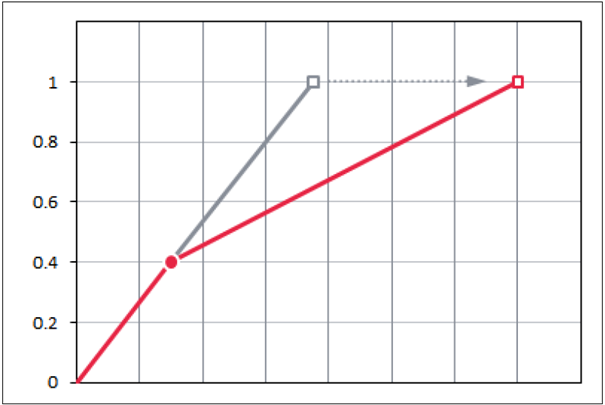Optimized Procedure

Division of the diverging increment without changes to the subsequent increments

• Greater effort (greater number of calculation runs necessary)
• Smaller number of increments (smaller calculation time)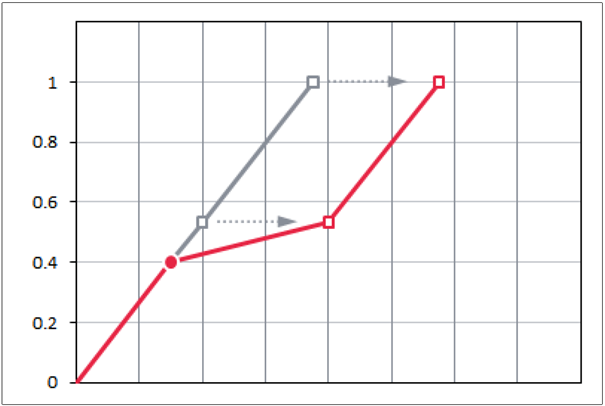Calculate the increase of the load factor between two increments.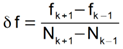Insert a new support point at the last converging increment.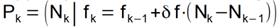Calculate the number of increments to introduce.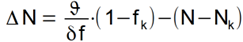Move the last support point.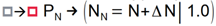Calculate the number of increments to introduce.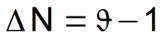Introduce and move a support point at the first diverging increment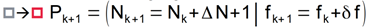Move all subsequent support points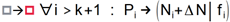• N = Number of increments
• Pk = (Nk | fk) last converging increment
• Pk-1 = (Nk-1 | fk-1) Support point before the last converging increment
• Pk+1 = (Nk+1 | fk+1) Support point after the last converging increment
• ϑ (z.B. ϑ = 5) Division ratio (ratio of slopes before and after division) a smaller ratio leads to a more optimized increment function but increases the necessary number of passes

A new calculation can produce the situations below.

• Calculation converges. Further optimization is not necessary.
• Calculation diverges in the same increment. Redivision of the segments, shallower slope of the load factor in the respective section.
• Calculation diverges in a later increment. Redivision of the segments, additional section of the curve with shallower slope.

### Example | Simple Procedure#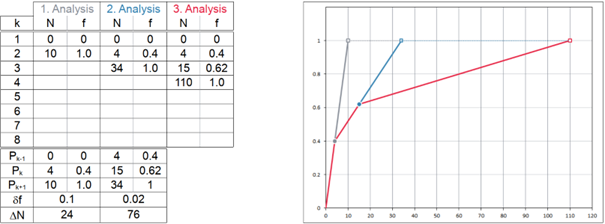### Example | Optimized Procedure#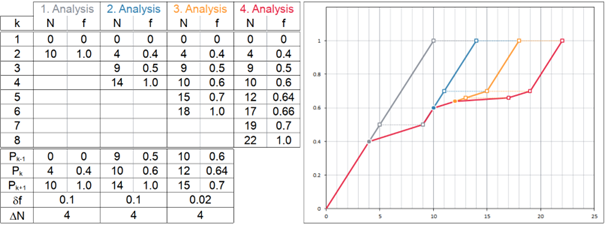### Summary#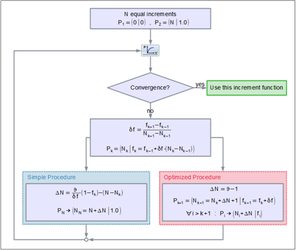Both procedures can be used to optimize a divergent calculation to meet the convergence criteria. But they are not the only procedures possible. Modified, refined, or completely different procedures can also produce good results.

Source: Ingware AG